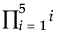Publication date: 04/12/2021

## Product

The Product() function is similar to Summation() except that it multiplies the body results rather than adding them. The syntax is the same as for Summation(). For example:

`p = Product( i = 1, 5 , i );`

returns 120, the result of 1*2*3*4*5.

In this example, the initial value of i is 1, the upper limit is 5, then all integer values of i up to 5 are multiplied.

Here is the equivalent in the Formula Editor:Want more information? Have questions? Get answers in the JMP User Community (community.jmp.com).